﻿ Inverse Trigonometric Function: Arccosine

# Inverse Trigonometric Function: Arccosine

LESSON READ-THROUGH (Part 1 of 2)
by Dr. Carol JVF Burns (website creator)
Follow along with the highlighted text while you listen!
• PRACTICE (online exercises and printable worksheets)

Before studying this section, you are encouraged to read Trying to ‘Undo’ Trigonometric Functions.

This section is a copy of Inverse Trigonometric Function: Arcsine, with appropriate changes.
If you've mastered the arcsine section, then this one should be quick and easy!

The arccosine function was informally introduced in Using the Law of Cosines in the SSS case (& Introduction to the Arccosine Function). It is made precise here.

 For a function to have an inverse, each output must have exactly one corresponding input. Thus, only one-to-one functions have inverses. The cosine function doesn't have a true inverse, because the cosine function is not one-to-one. So, to try and define an ‘inverse cosine function’, we do the best we can. We throw away most of the cosine curve, leaving us with a piece that has three properties: the piece is one-to-one (and hence has an inverse) the piece covers all the outputs from the cosine function (the interval $\,[-1,1]\,$) the piece is close to the origin The cosine curve has two pieces that meet all three requirements: the green piece shown at right the red piece shown at right The green piece is nicer, because it has positive $x$-values. Positive numbers are easier to work with than negative numbers. This green piece is the restriction of the cosine curve to the interval $\,[0,\pi]\,.$ The function that the mathematical community calls ‘the inverse cosine function’ is not actually the inverse of the cosine function, because the cosine function doesn't have a true inverse. Instead, the ‘inverse cosine function’ is the inverse of this green piece of the cosine curve. Several Cycles of the Graph of the Cosine Function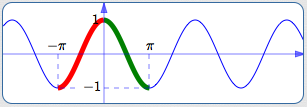The cosine function isn't one-to-one; it doesn't pass a horizontal line test. So, it doesn't have a true inverse. To define an ‘inverse cosine function’,we do the best we can. Throw away most of the curve—leave only the green part. This green part is one-to-one. This green part does have an inverse. The inverse of this green part is what the mathematical community calls ‘the inverse cosine function’.

The arccosine function (precise definition below) is the best we can do in trying to get an inverse of the cosine function.
The arccosine function is actually the inverse of the green piece shown above!

Here's a ‘function box’ view of what's going on:
 The cosine function takes a real number as an input. It gives an output in the interval $\,[-1,1]\,.$ For example (as below), the output $\,0.5\,$ might come from the cosine function.When we try to use the cosine function box ‘backwards’, we run into trouble. The output $\,0.5\,$ could have come from any of the inputs shown.However, when we use the green piece of the cosine curve,the problem is solved! Now, there's only one input that works. (It's the value of the green $\,\color{green}{x}\,.$ ) Observe that $\,\color{green}{x}\,$ is in the interval $\,[0,\pi]\,.$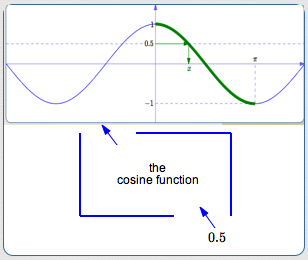It's a bit of a misnomer, but the arccosine function (precise definition below) is often referred to as the ‘inverse cosine function’.
A better name would be something like ‘the inverse of an appropriately-restricted cosine function’.
(It's no surprise, however, that people don't say something that long and cumbersome.)

So, what exactly is $\,\arccos 0.5\,$?

$\,\arccos 0.5\,$ is the number in the interval $\,[0,\pi]\,$ whose cosine is $\,0.5\,$

## What exactly IS $\,\arccos x\,$?

More generally, let $\,x\,$ be any number in the interval $\,[-1,1]\,.$
Then:

$\,\arccos x\,$ is the number in the interval $\,[0,\pi]\,$ whose cosine is $\,x\,$

In my own mind (author Dr. Carol Burns speaking here), the words I say are:

$\,\arccos x\,$ is the number between $\,0\,$ and $\,\pi\,$ whose cosine is $\,x\,$
I personally know the endpoints are included, so this doesn't confuse me.
However, the word ‘between’ is ambiguous—it can include the endpoints or not, depending on context.
It can be clarified by saying:
$\,\arccos x\,$ is the number between $\,0\,$ and $\,\pi\,$ (including the endpoints) whose cosine is $\,x\,$
... but then it loses its simplicity. Ah—issues with language. Choose words that work for you!

## Precise Definition of the Arccosine Function

The precise definition of the arccosine function follows.
It can look a bit intimidating—the notes following the definition should help.

DEFINITION the arccosine function, denoted by  $\,\arccos\,$  or  $\,\cos^{-1}\,$
Let $\,-1 \le x\le 1\,.$

Using the notation ‘$\,\arccos\,$’ for the arccosine function: $$\cssId{s69}{y = \arccos x}\ \ \ \ \ \cssId{s70}{\text{if and only if}}\ \ \ \ \cssId{s71}{\bigl(\ \cos y = x\ \ \text{AND}\ \ 0 \le y\le \pi\ \bigr)}$$ Using the notation ‘$\,\cos^{-1}\,$’ for the arccosine function: $$\cssId{s73}{y = \cos^{-1} x}\ \ \ \ \ \cssId{s74}{\text{if and only if}}\ \ \ \ \cssId{s75}{\bigl(\ \cos y = x\ \ \text{AND}\ \ 0 \le y\le \pi\ \bigr)}$$

## Notes on the Definition of the Arccosine Function:

• UNDERSTANDING THE DEFINITION:
The definition precisely answers the question: ‘What is the number $\,\arccos x\,$?’
Here's how:
• Recall that ‘ if and only if ’ means the same thing as is equivalent to.
Equivalent sentences have identical truth values:
if one is true, so is the other;   if one is false, so is the other.
• Thus, if the sentence ‘$\,y = \arccos x\,$’ is true,
then the compound sentence   ‘$\,\cos y = x\ \ \text{AND}\ \ 0 \le y\le \pi\,$’   must also be true.
• If   ‘$\,y = \arccos x\,$’   is true, then ‘$\,y\,$’ is just another name for ‘$\,\arccos x\,$’.
• If   ‘$\,y = \arccos x\,$’   is true, then two things must be true about $\,\arccos x\,$ (i.e., $\,y\,$):
• $\,\cos \overbrace{y}^{\arccos x} = x\,$:   its cosine must be $\,x\,$
• $\,0 \le \overbrace{y}^{\arccos x}\le \pi\,$:   it must be in the interval $\,[0,\pi]\,$
• Combining these ideas: $$\cssId{s96}{\overbrace{\strut y = \arccos x}^{\text{How does a number get to be called \,\arccos x\,?}}}\ \ \ \ \ \cssId{s97}{\overbrace{\strut \text{if and only if}}^{\text{The answer is:}}}\ \ \ \ \bigl(\ \cssId{s98}{\overbrace{\strut \cos y = x}^{\text{by having its cosine equal to \,x\,}}}\ \ \cssId{s99}{\overbrace{\strut \text{AND}}^{\text{and}}}\ \ \cssId{s100}{\overbrace{\strut \ 0 \le y\le \pi\ \ }^{\text{by being in the interval \strut \,[0,\pi]\,}}}\ \bigr)$$
• That is:
$\,\arccos x\,$ is the number in the interval $\,[0,\pi]\,$ whose cosine is $\,x\,$

• FORMAL NAME VERSUS FUNCTION NOTATION ABBREVIATIONS:
The formal name of the function being discussed in this section is ‘arccosine’.
It is pronounced arc-CO-sine (with a long ‘i’ in ‘sine’).

When using function notation, ‘arccosine’ is abbreviated as ‘$\,\arccos\,$’.
It is pronounced the same as ‘arccosine’.
Thus, ‘$\,\arccos x\,$’ is read aloud as ‘arccosine of $\,x\,$’.

An alternate notation for the arccosine function is ‘$\,\cos^{-1}\,$’.
This alternate notation is modeled on the standard notation for inverse functions:
if $\,f\,$ is one-to-one, then its inverse is called $\,f^{-1}\,.$

The function notation ‘$\,\cos^{-1} x\,$’ can be read aloud as ‘arccosine of $\,x\,$’ or ‘the inverse cosine of $\,x\,$’.
DON'T read ‘$\,\cos^{-1} x\,$’ as ‘cosine to the negative one of $\,x\,$’!
There is no reciprocal operation going on here—it's just standard notation for an inverse function.
• CONVENTION FOR MULTI-LETTER FUNCTION NAMES:
Since both ‘$\,\arccos\,$’ and ‘$\,\cos^{-1}\,$’ are multi-letter function names, the standard convention applies:
the parentheses that typically hold the input can be removed, if there is no possible confusion about order of operations.

Thus, you usually see   ‘$\,\arccos x\,$’   and   ‘$\,\cos^{-1} x\,$’   (no parentheses),
instead of the more cumbersome   ‘$\,\arccos (x)\,$’   and   ‘$\,\cos^{-1} (x)\,$’   (with parentheses).
• FUNCTION NAME VERSUS OUTPUT FROM THE FUNCTION:
The function name is ‘$\,\arccos\,$’.
The number ‘$\,\arccos x\,$’ is the output from the function ‘$\,\arccos\,$’ when the input is $\,x\,.$

Similarly, the function name is ‘$\,\cos^{-1}\,$’.
The number ‘$\,\cos^{-1} x\,$’ is the output from the function ‘$\,\cos^{-1}\,$’ when the input is $\,x\,.$
• PREFERRED NOTATION:
Since the cosine function does not have a true inverse,
this author believes the notation ‘$\,\cos^{-1}\,$’ is misleading and lends itself to errors.
This author strongly prefers the notation ‘$\,\arccos\,$’.
Inputs to trigonometric functions can be viewed as real numbers (radian measure) or degrees.
For example, $\,\cos \pi = -1\,$:   here, $\,\pi\,$ is radian measure.
Equivalently, $\,\cos 180^\circ = -1\,$:   here, $\,180^\circ\,$ is degree measure.

Here's what the definition of arccosine looks like, using degree measure instead of radian measure: $$\cssId{sb31}{y = \arccos x}\ \ \ \ \ \cssId{sb32}{\text{if and only if}}\ \ \ \ \cssId{sb33}{\bigl(\ \cos y = x\ \ \text{AND}\ \ 0^\circ \le y\le 180^\circ\ \bigr)}$$
$\,\arccos x\,$ is the angle in the interval $\,[0^\circ,180^\circ]\,$ whose cosine is $\,x\,$
• CALCULATOR SKILLS:
If a calculator is in degree mode, then $\,\arccos x\,$ is reported in degrees.
If a calculator is in radian mode, then $\,\arccos x\,$ is reported in radians.
• GRAPH OF THE ARCCOSINE FUNCTION:
For a one-to-one function $\,f\,,$ the graph of its inverse, $\,f^{-1}\,,$ is found by reflecting the graph of $\,f\,$ about the line $\,y = x\,.$
Below, this technique is used to construct the graph of the arccosine function:

 Here's the piece of the cosine curve that is used to define the arccosine function: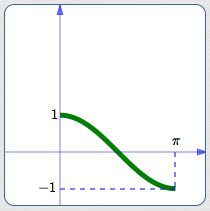domain:   $\,[0,\pi]\,$ range:   $\,[-1,1]\,$ Here's the same curve, together with its reflection about the line $\,\color{red}{y = x}\,$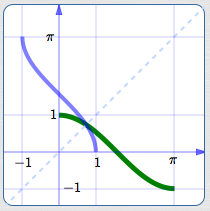The graph of the arccosine function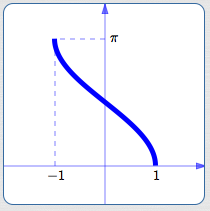domain:   $\,[-1,1]\,$ range:   $\,[0,\pi]\,$ Notice that the domain and range of a function and its inverse are switched! The domain of one is the range of the other. The range of one is the domain of the other.
• RELATIONSHIP BETWEEN THE COSINE AND ARCCOSINE FUNCTIONS:
For a one-to-one function $\,f\,$ and its inverse $\,f^{-1}\,,$ there is a simple ‘undoing’ relationship between the two:
• $f^{-1}\bigl(f(x)\bigr) = x\,$ for all $\,x\,$ in the domain of $\,f\,$:
the function $\,f\,$ does something, and $\,f^{-1}\,$ undoes it
• $f\bigl(f^{-1}(x)\bigr) = x\,$ for all $\,x\,$ in the range of $\,f\,$:
the function $\,f^{-1}\,$ does something, and $\,f\,$ undoes it
Since the cosine and arccosine functions are not true inverses of each other,
the relationship between them is a bit more complicated.

 Here's the direction where they do ‘undo’ each other nicely: start with a number, first apply the arccosine function, then apply the cosine function, and end up right where you started. Here are the details: For all $\,x\in [-1,1]\,,$ $$\cssId{sb66}{\cos(\arccos x) = x}$$ start with $\,\color{red}{x}\in [-1,1]\,$ the arccosine function takes $\,\color{red}{x}\,$ to $\,\color{green}{\arccos x}\,$ in the interval $\,[0,\pi]\,$ the cosine function takes $\,\color{green}{\arccos x}\,$ back to $\,\color{red}{x}\,$Here's the direction where they don't necessarily ‘undo’ each other nicely: start with a number, first apply the cosine function, then apply the arccosine function. If the number you started with is outside the interval $\,[0,\pi]\,,$ then you don't end up where you started! Here are the details: For all $\,x\in [0,\pi]\,,$ $$\cssId{sb76}{\arccos(\cos x) = x}$$ (See the top graph at right.) For all $\,x\not\in [0,\pi]\,,$ $$\cssId{sb79}{\arccos(\cos x) \ne x}$$ (See the bottom graph at right.)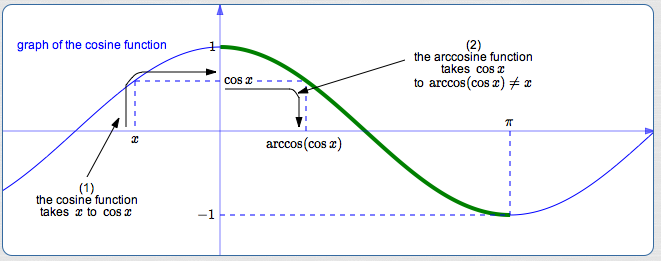## Example: Find the Exact Value of $\,\arccos(-0.5)\,$(use both the unit circle and a special triangle)

 Using the degree definition: $\,\arccos (-\frac 12)\,$ is the angle between$\,0^\circ\,$ and $\,180^\circ\,$ whose cosine is $\,-\frac 12\,.$ cosine is the $x$-value of points on the unit circle. Draw a unit circle. Mark $\,-\frac 12\,$ on the $x$-axis. Mark the unique angle between $\,0^\circ\,$ and $\,180^\circ\,$ that has this cosine value. This (positive) angle is $\,\arccos(-\frac 12)\,.$ Does any special triangle tell us an acute angle whose cosine is $\,\frac12\,$? Yes! The cosine of $\,60^\circ\,$ is $\,\frac 12\,.$ Thus, the reference angle is $\,\color{red}{60^\circ}\,,$ as shown. Thus, $\,\arccos(-\frac 12) = 180^\circ - 60^\circ = 120^\circ\,.$ Using radian measure: $$\displaystyle\,\cssId{sb98}{\arccos(-\frac 12) = \frac{2\pi}3}\,$$Master the ideas from this section